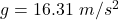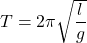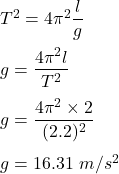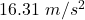I need this now On the planet Xenos, an astronaut observes that a 2.0 m long pendulum has a period of 2.2 s. What is the f

Question

I need this now

On the planet Xenos, an astronaut observes that a 2.0 m long pendulum has a period of 2.2 s.
What is the free-fall acceleration on Xenos? *

in progress 0
6 months 2021-07-21T16:25:53+00:00 1 Answers 0 views 0Explanation:

Given that,

The length of the pendulum, l = 2 m

The period of the pendulum, T = 2.2 s

The formula for the time period of a pendulum is given by :orSo, the free fall acceleration is.